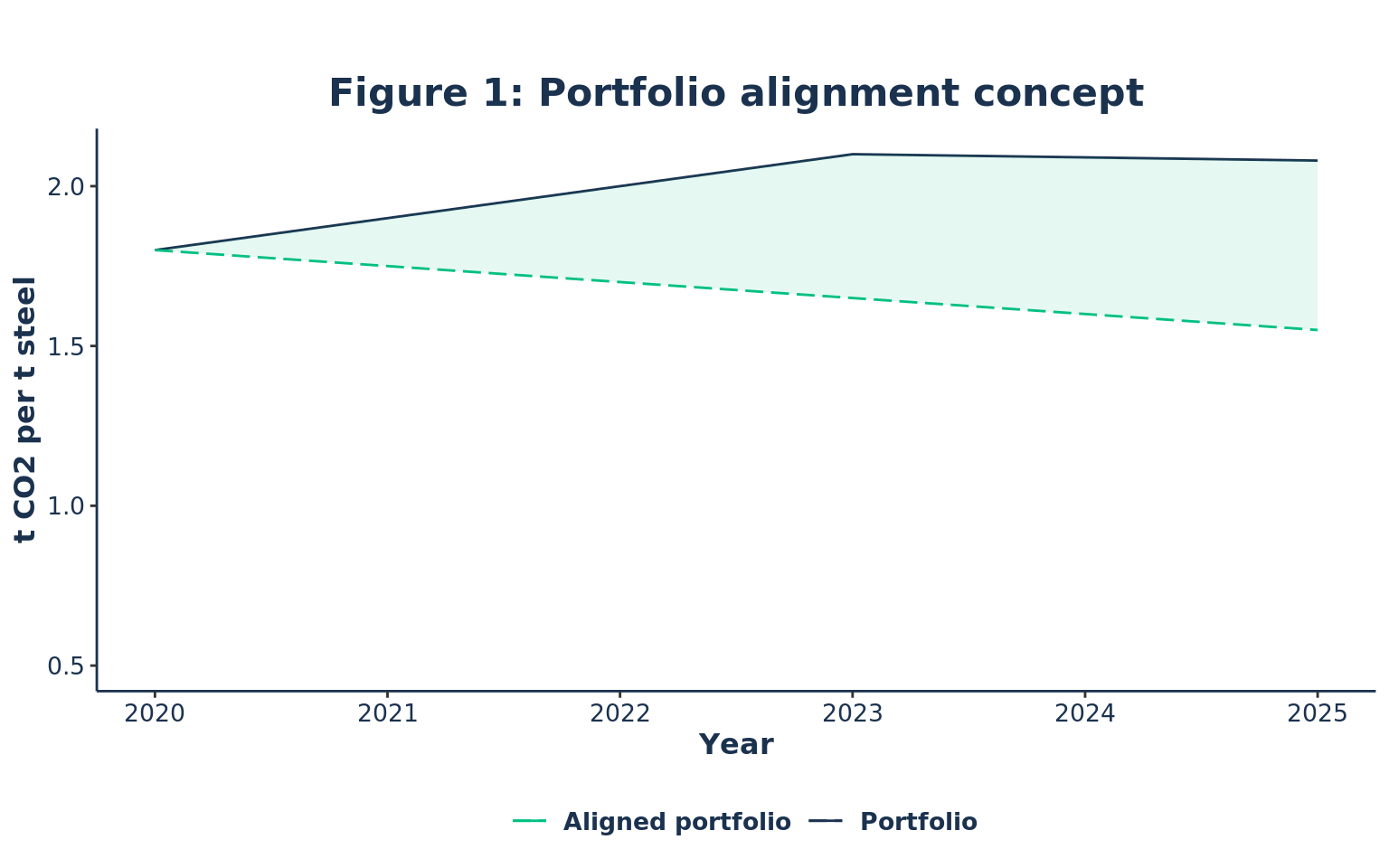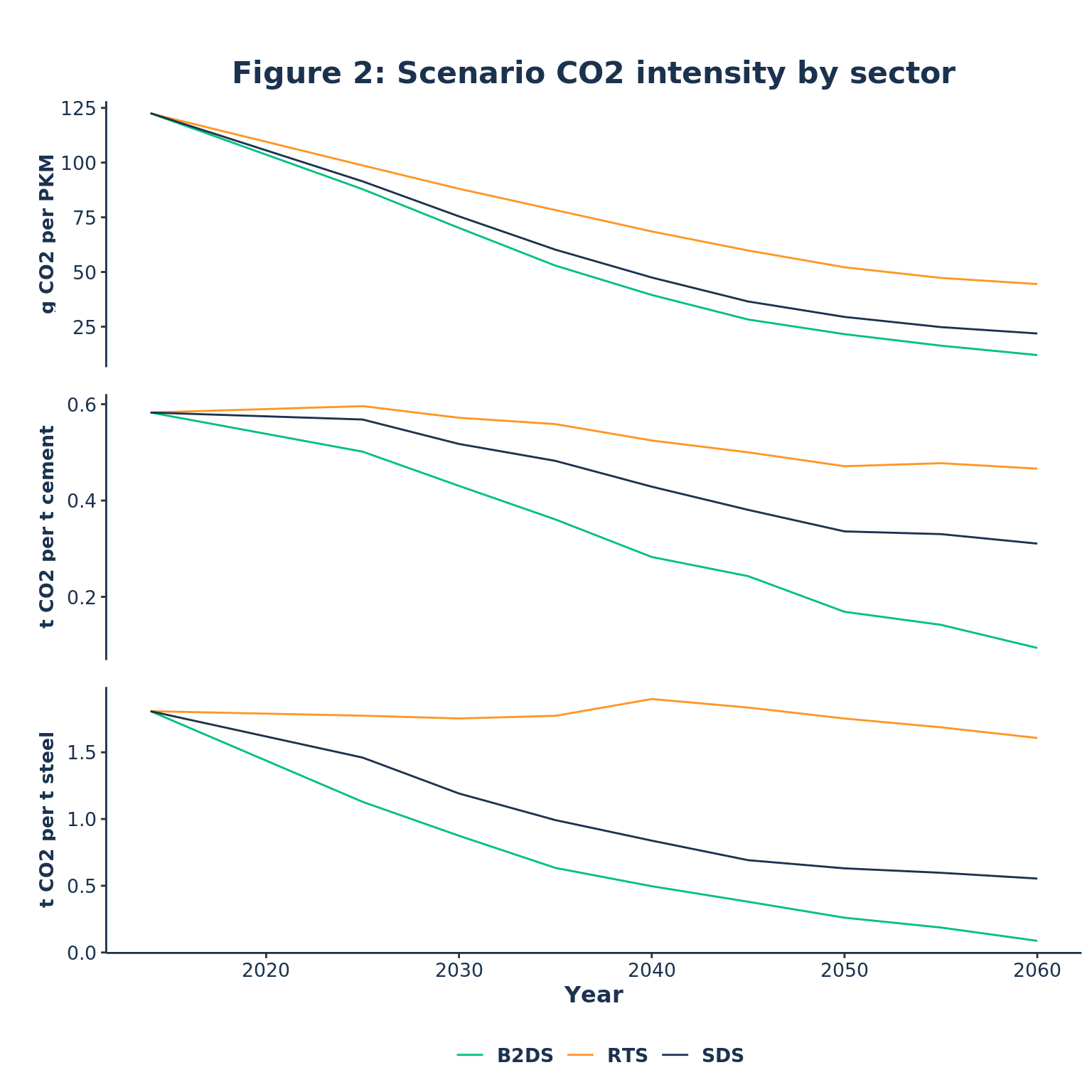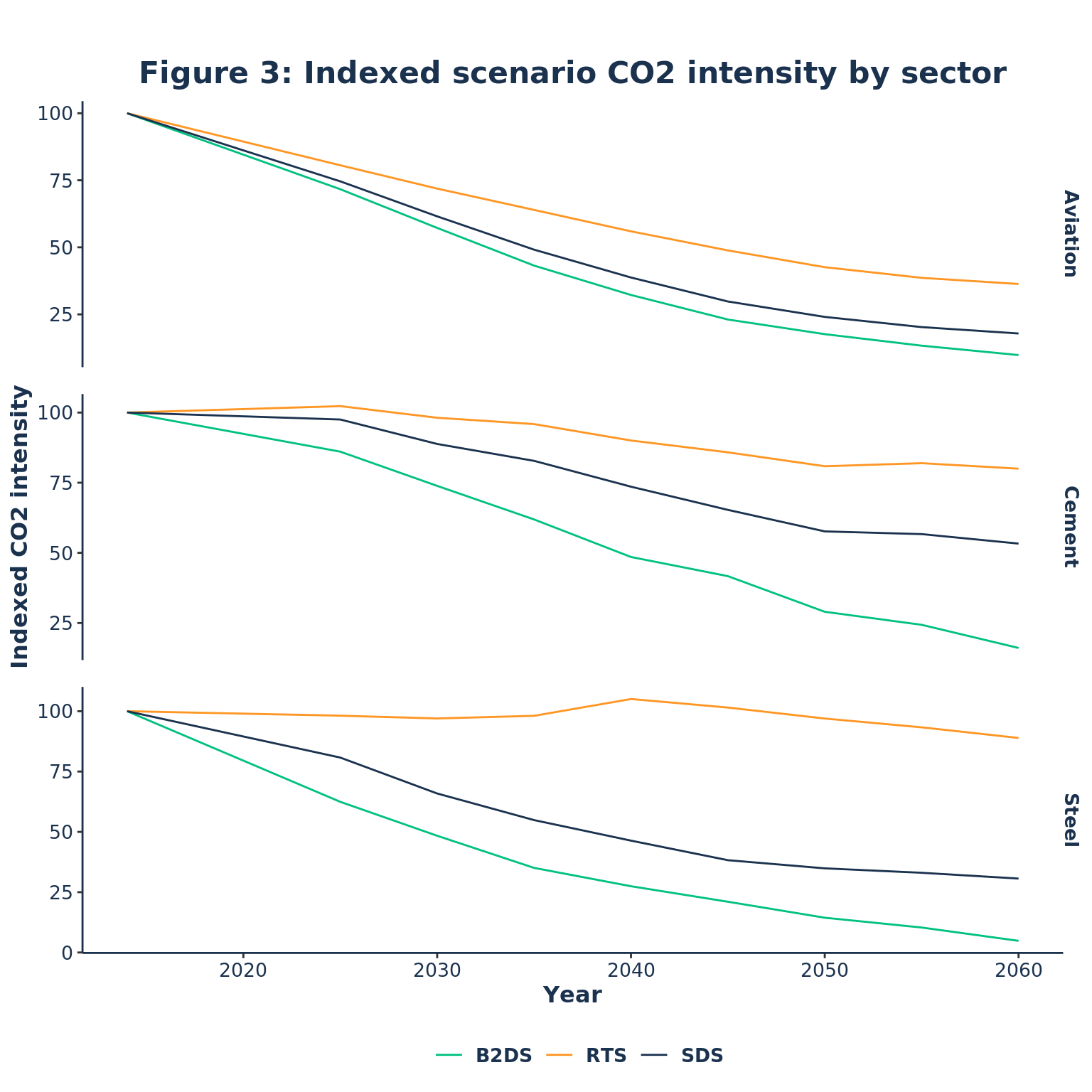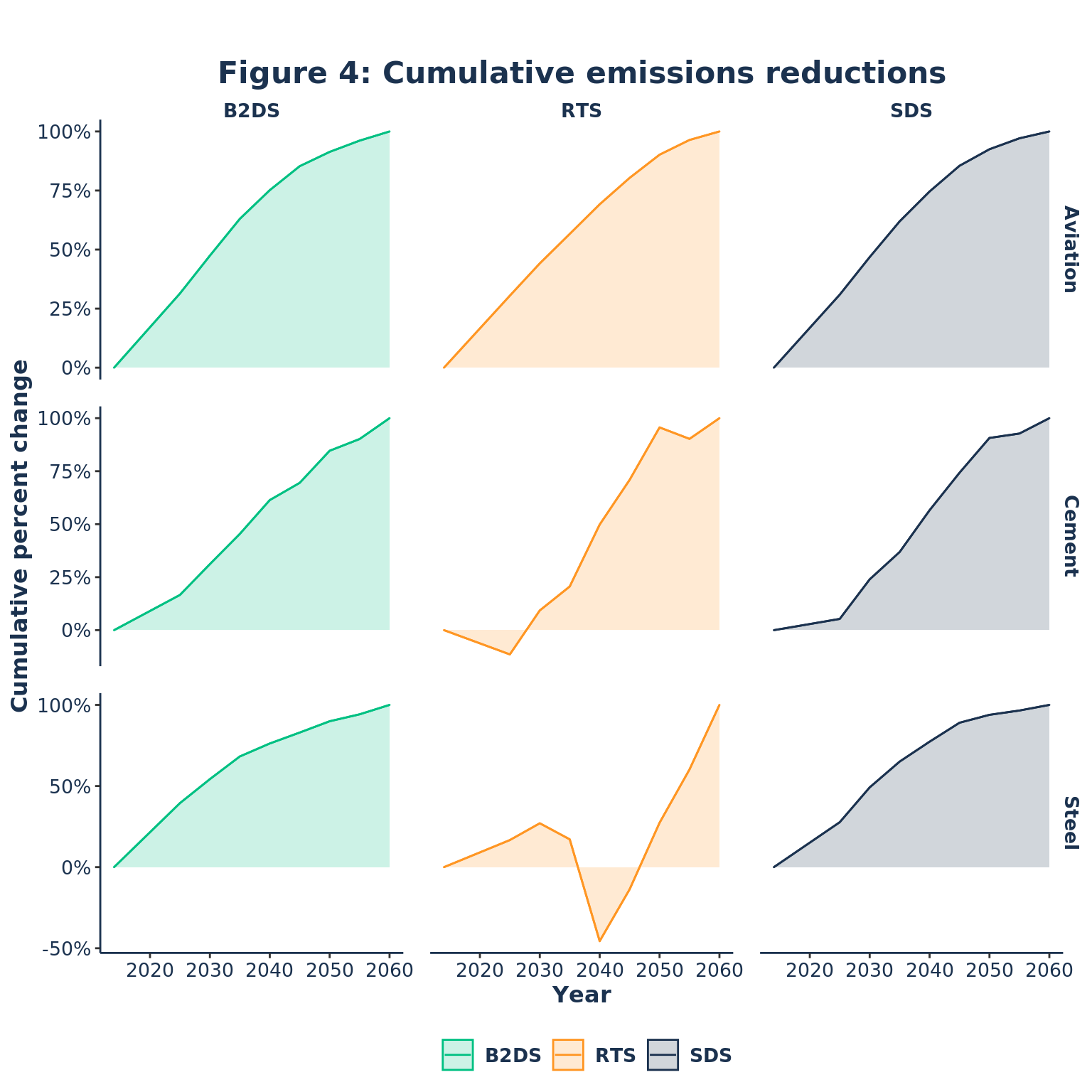# When Scenarios and Reality Diverge

A deep dive into IEA scenarios.

Vincent Jerosch-Herold https://github.com/vintented
07-24-2020

# Context

A scenario lays-out an ‘evolution’ of the world. In financial markets this evolution reflects current and future capital flows within and across sectors as high-carbon technologies are replaced with low-carbon. Scenario analysis is simply the process of scaling and allocating scenario macro changes to the micro — the economic activities linked to financial portfolios. The scenario alignment of a portfolio is simply the divergence overtime between the portfolio’s baseline (e.g. the portfolio’s $$CO_2$$ intensity over the analysis time-horizon) and the scaled ambition of the scenario (see Figure 1).The scaled trajectory of a scenario suggests that a portfolio is consistent with a scenario when the evolution of the indicator (e.g. $$CO_2$$ intensity) is consistent with what the scenario prescribes. What this entails in practice is from the portfolio’s current baseline (e.g. $$CO_2$$ intensity in 2020), indexing the scenario changes from that baseline over the entire scenario’s time horizon. This ensures the differing scopes of the scenario (i.e. macro-economy model) and portfolio (i.e. a specific subset of macro-economic activities) can be bridged by ensuring equal ambition regardless of scope. However, a portfolio’s current baseline is not always consistent with the evolution of a scenario — the International Energy Agency’s (IEA) Energy Technology Perspective (ETP) illustrates this point apptly.(IEA 2017)

ETP 2017 roadmaps a global energy transition that begins in 2014 along three scenario sets, RTS (Reference Technology Scenario), SDS (Sustainable Development Scenario), and B2DS (Beyond 2 Degrees Scenario) (in order of ambition).(IEA 2017) Each scenario proscribes a set of socioeconomic developments informed by the varying potential of low-carbon technologies to reduce emissions across sectors. These reductions are mapped in terms of the shift from high to low-carbon technologies in units of production or capacity (e.g. TWh, tonnes of steel, etc.).

However, if the current state of the world is any indication, a global, cross-sector energy transition has yet take place.(IPCC 2018) To illustrate this point, the IEA assumes that in 2020 the direct $$CO_2$$ intensity of global steel production has declined by ~20% under ETP’s B2DS.(IEA 2017) In reality, the global $$CO_2$$ intensity of steel production from 2014 to 2020 has not significantly deviated from $$1.85 \ kg \ CO_2 \ per \ kg \ steel$$.(World Steel Association 2019) In effect, The evolution of the scenario and the macro-economy differ, and the longer global emissions continue to rise, the greater the disconnect. And this temporal and evolutionary disconnect between the portfolio (a subset of macro-economic activities) and scenario has implications on the scaled scenario ambition assigned to the portfolio.

# Data preperation

The input data is simply the CO2 intensity for Cement (scope 1 & 2), Steel (scope 1 & 2), and Aviation (scope 1) from ETP 2017.(IEA 2017)


# packages
library(gt)
library(tidyverse)
# themes
source("ggplot_themes.R")
# data
# functions
index_market_to_scenario <- function(
scenario,
aviation_intensity = 87.9,
steel_intensity = 1.8,
cement_intensity = 0.6
) {
year_seq <- unique(scenario\$year)
lagged_scenario <- map(
2:length(year_seq),
function(i) {
scenario %>%
select(
scenario,
sector,
year,
emission_factor
) %>%
group_by(
scenario,
sector
) %>%
mutate(
index = ifelse(!year %in% year_seq[1:(i-1)], emission_factor, NA_integer_) / sum(ifelse(year == year_seq[i], emission_factor, 0)),
emission_factor_ald = case_when(
sector == "aviation" ~ aviation_intensity * index,
sector == "steel" ~ steel_intensity * index,
sector == "cement" ~ cement_intensity * index
),
lag = paste("Lag", (i-1))
) %>%
filter(!is.na(index)) %>%
rename(emission_factor_scenario = emission_factor) %>%
ungroup()
}
)
# bind lags together
lagged_scenario %>%
bind_rows()
}
data %>%
mutate(
scenario = toupper(scenario),
sector = stringr::str_to_title(sector)
)
}

table_data <- etp2017_sda_targets %>%
group_by(
sector,
emission_factor_unit
) %>%
summarise(
Min = min(emission_factor),
Max = max(emission_factor)
) %>%
ungroup()

table_data %>%
rename(
Sector = sector,
Unit = emission_factor_unit
) %>%
gt() %>%
theme_2dii_gt() %>%
title = "Table 1: A brief overview of units",
subtitle = "CO2 intensity"
)
Table 1: A brief overview of units
CO2 intensity
Sector Unit Min Max
Aviation grams CO2 (scope 1) per passenger-km 12.02445334 122.5599610
Cement tonnes CO2 (scope 1 & 2) per tonne cement 0.09386214 0.5959728
Steel tonnes CO2 (scope 1 & 2) per tonne crude steel 0.08728846 1.8990379

Scope 2 emissions are calculated using the average emissions intensity of global power generation and electricity energy demand from the Steel and Cement sectors. For Aviation, only the emissions from the combustion of Jet-fuel (scope 1) are considered.

E.g. Steel scope 2 emissions: $Power \ Emissions \ _{CO_2 \ Mt} = \frac{Electricity \ Demand \ _{PJ}} {3600} \ * Power \ Emissions \ Intensity \ _{\frac{CO_2 \ {Mt}} {MWh}}$

With the electricy energy demand and electricity CO2 intensity, the scope 2 emissions intensity per unit of output (e.g. tonnes of Steel) can be calculated. Scope 1 emissions are calculated by dividing direct CO2 emissions per unit of product.

E.g. Steel CO2 intensity: $Emissions \ Intensity \ _{\frac{CO_2 \ {Mt}} {Crude \ Steel \ Mt }} = \sum {\frac{Direct \ Emissions \ _{CO_2 \ Mt}} {Production \ _{Crude \ Steel \ Mt}}}$

# Data comparison

Perhaps it nice to begin by simply comparing the $$CO_2$$ intensity across scenarios and sectors. Figure 2 illustrates the previously mentioned difference in terms of ambition across the three ETP scenario sets with the B2DS following the most ambitious emissions intensity reduction path and the RTS following the least ambitious path.(IEA 2017)


etp2017_sda_targets %>%
ggplot(aes(x = year, y = emission_factor, color = scenario, group = scenario)) +
geom_line() +
labs(x = "Year", y = "", title = "Figure 2: Scenario CO2 intensity by sector") +
scale_color_manual(values = c(primary_green, primary_orange, primary_blue)) +
facet_grid(
rows = vars(sector),
switch = 'y',
scales = "free_y",
labeller = as_labeller(c(Steel = "t CO2 per t steel", Cement = "t CO2 per t cement", Aviation = "g CO2 per PKM"))
) +
theme_2dii_ggplot() +
theme(strip.placement = 'outside')To compare apples to apples Figure 3 illustrates the difference across scenarios and sectors indexed to the base year 2014.


# calculate index
chart_data <- etp2017_sda_targets %>%
group_by(
scenario,
sector
) %>%
mutate(index = 100 * (emission_factor / sum(ifelse(year == min(year), emission_factor, 0)))) %>%
ungroup()
# plot data
chart_data %>%
ggplot(aes(x = year, y = index, color = scenario, group = scenario)) +
geom_line() +
labs(x = "Year", y = "Indexed CO2 intensity", title = "Figure 3: Indexed scenario CO2 intensity by sector") +
scale_color_manual(values = c(primary_green, primary_orange, primary_blue)) +
facet_grid(rows = vars(sector), scales = "free_y") +
theme_2dii_ggplot()Having compared how the $$CO_2$$ intensity evolves over the scenario’s time horizon, it is perhaps of interest to understand the distribution of these cumulative changes. Figure 4 shows the annual rate of change in $$CO_2$$ intensity as a percent of the total change in $$CO_2$$ intensity over the scenario’s time horizon. Depending on the scenario’s ambition and the sector, these emissions intensity reductions vary in distribution. Both B2DS and SDS follow relatively similar distributions with the rate change following a comparable trajectory until mid-century when the curve flattens out. This trend reflects the “hard to decarbonize” nature of industrial processes and Aviation, where progress will likely be incremental, unlike transformational changes in other sectors like Power.


# calculate metrics
chart_data <- etp2017_sda_targets %>%
group_by(
scenario,
sector
) %>%
mutate(
total_change = first(emission_factor) - last(emission_factor),
annual_change =  lag(emission_factor, n = 1L) - emission_factor,
percent_of_total_change = annual_change / total_change
) %>%
ungroup()
# calculate cumsum
chart_data <- chart_data %>%
filter(!is.na(percent_of_total_change)) %>%
group_by(
scenario,
sector
) %>%
arrange(year, by_group = TRUE) %>%
mutate(cumsum_of_percent_of_total_change = cumsum(percent_of_total_change)) %>%
distinct(
year,
scenario,
sector,
cumsum_of_percent_of_total_change
) %>%
ungroup()
# create baseline
chart_data <- chart_data %>%
distinct(
scenario,
sector
) %>%
mutate(
year = 2014,
cumsum_of_percent_of_total_change = 0
) %>%
bind_rows(chart_data)
# plot chart
chart_data %>%
ggplot(aes(x = year, y = cumsum_of_percent_of_total_change, fill = scenario, color = scenario)) +
geom_area(alpha = 0.2) +
geom_line() +
theme_2dii_ggplot() +
scale_color_manual(values = c(primary_green, primary_orange, primary_blue)) +
scale_fill_manual(values = c(primary_green, primary_orange, primary_blue)) +
scale_y_continuous(labels = scales::label_percent()) +
facet_grid(rows = vars(sector), cols = vars(scenario), scales = "free_y") +
labs(x = "Year", y = "Cumulative percent change", title = "Figure 4: Cumulative emissions reductions")Inspired by a similar chart from the SENSE project, Figure 5 shows the change in $$CO_2$$ intensity trajectories over 5-year increments across sectors and scenarios.(PIK, IIASA, and SEI 2020) If each line represents a hypotenuse with the x and y-axis representing the adjacent and opposite sides, the longer the hypotenuse, the greater the emission intensity reductions over the 5-year increment.


# create year intervals
chart_data <- etp2017_sda_targets_interprolated %>%
mutate(
year_range = case_when(
between(year, 2014, 2025) ~ "2014-2025",
between(year, 2025, 2030) ~ "2025-2030",
between(year, 2030, 2035) ~ "2030-2035",
between(year, 2035, 2040) ~ "2035-2040",
between(year, 2040, 2045) ~ "2040-2045",
between(year, 2045, 2050) ~ "2045-2050",
between(year, 2050, 2055) ~ "2050-2055",
between(year, 2055, 2060) ~ "2055-2060"
)
)
# calculate first last ratios
chart_data <- chart_data %>%
group_by(
scenario,
sector,
year_range
) %>%
mutate(
start_value = first(emission_factor) / last(emission_factor),
end_value = last(emission_factor) / first(emission_factor)
) %>%
distinct(
scenario,
sector,
year_range,
start_value,
end_value
) %>%
ungroup()
# pivot wider
chart_data <- chart_data %>%
pivot_longer(
cols = c("start_value", "end_value"),
names_to = "start_end",
values_to = "trajectory"
)
# plot chart
chart_data %>%
theme(axis.text.x = element_blank(), axis.ticks.x = element_blank(), axis.line.x = element_blank(), axis.title.x = element_blank(), strip.text.x = element_text(family="GT America", color=primary_blue, size = 10, face = "plain"), panel.spacing = unit(0.1, "cm"))